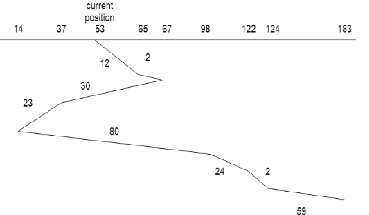# UGC NET COMPUTER SCIENCE SOLVED PAPERS 2017-19 - UGC NET Computer Science November 2017 Paper 3

>>>>>>>>UGC NET Computer Science November 2017 Paper 3

• A

(a) is true; (b) is false• B

(a) is false; (b) is true• C

Both (a) and (b) are true• D

Both (a) and (b) are false• Option : A
• Explanation :
(a)
(i) The Many - to - one model maps many user threads to one kernel thread
(ii) The one - to - one model maps one user thread to one kernel thread
(iii) The many - to - many model maps many user threads to smaller or equal kernel threads
(b)
(i) Many - to - one model maps many kernel threads to one user thread
(ii) One - to - one model maps one kernel thread to one user thread
(iii) Many - to - many model maps many kernel threads to smaller or equal user threads All statement are correct.
So, option (A) is correct.

If we implement Deadlock detection algorithm we claim that system is __________.
(1) Semaphore
(2) Deadlock state
(3) Circular wait
(4) Not in deadlock state

• A

(1), (2) and (3)• B

(1) and (3)• C

(3) and (4)• D

All are correct.• A

224• B

248• C

236• D

240• Option : C
• Explanation :Now add all seek time: 12+2+30+23+84+24+2+59=236 So, option (C) is correct.

• A

Producer - consumer problem• B

Reader - writer problem• C

Dining Philosophers problem• D

Both (2) and (3)• Option : A
• Explanation :
The bounded buffer problem is also known as producer-consumer problem.
So, option (A) is correct.

• A

Simple reflex agent• B

Model based agent• C

Learning agent• D

Utility based agent• Option : D
• Explanation :
Utility based agent deals with happy and unhappy state in artificial intelligence (AI). So, option (D) is correct.